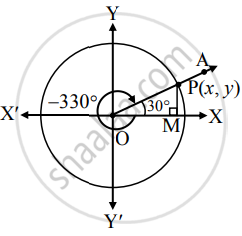# Answer the following: Find the trigonometric functions of : −330° - Mathematics and Statistics

Sum

Find the trigonometric functions of :

−330°

#### Solution

Angle of measure (– 330°) :Let m∠XOA = −330°

Its terminal arm (ray OA) intersects the standard unit circle at P(x, y).

Draw seg PM perpendicular to the X-axis.

∴ ΔOMP is a 30° – 60° – 90° triangle.

OP = 1

OM = sqrt(3)/2"OP"

= sqrt(3)/2(1)

= sqrt(3)/2

PM = 1/2"OP"

= 1/2(1)

= 1/2

Since point P lies in the 1st quadrant,

x > 0, y > 0

∴ x = OM = sqrt(3)/2 and y = "PM" = 1/2

∴ P ≡ (sqrt(3)/2, 1/2)

sin (−330°) = y = 1/2

cos (−330°) = x = sqrt(3)/2

tan (−330°) = y/x = (1/2)/(sqrt(3)/2) = 1/sqrt(3)

cosec (−330°) = 1/y = 1/((1/2)) = 2

sec (−330°) = 1/x = 1/((sqrt(3)/2)) = 2/sqrt(3)

cot (−330°) = x/y = (sqrt(3)/2)/((1/2)) = sqrt(3)

Concept: Trigonometric Functions of Specific Angles
Is there an error in this question or solution?

#### APPEARS IN

Balbharati Mathematics and Statistics 1 (Arts and Science) 11th Standard Maharashtra State Board
Chapter 2 Trigonometry - 1
Miscellaneous Exercise 2 | Q II. (1) l. | Page 33